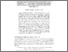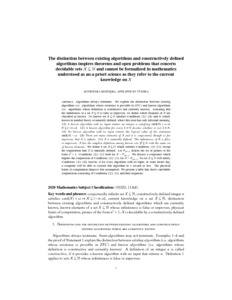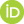# The distinction between existing algorithms and constructively defined algorithms inspires theorems and open problems that concern decidable sets X⊆N and cannot be formalized in mathematics understood as an a priori science as they refer to the current knowledge on X

Kozdęba, Agnieszka and Tyszka, Apoloniusz (2021) The distinction between existing algorithms and constructively defined algorithms inspires theorems and open problems that concern decidable sets X⊆N and cannot be formalized in mathematics understood as an a priori science as they refer to the current knowledge on X. [Preprint]There is a more recent version of this item available.Preview
Text
ak_at.pdf

## Abstract

Algorithms always terminate. We explain the distinction between existing algorithms (i.e. algorithms whose existence is provable in ZFC) and known algorithms (i.e. algorithms whose definition is constructive and currently known). Assuming that the infiniteness of a set X⊆N is false or unproven, we define which elements of X are classified as known. No known set X⊆N satisfies Conditions (1)-(4) and is widely known in number theory or naturally defined, where this term has only informal meaning. *** (1) A known algorithm with no input returns an integer n satisfying card(X)<ω ⇒ X⊆(-∞,n]. (2) A known algorithm for every k∈N decides whether or not k∈X. (3) No known algorithm with no input returns the logical value of the statement card(X)=ω. (4) There are many elements of X and it is conjectured, though so far unproven, that X is infinite. (5) X is naturally defined. The infiniteness of X is false or unproven. X has the simplest definition among known sets Y⊆N with the same set of known elements. *** We define a set X⊆N which satisfies Conditions (1)-(5) except the requirement that X is naturally defined. Let P(n^2+1) denote the set of primes of the form n^2+1. Conditions (2)-(5) hold for X=P(n^2+1). We discuss a conjecture which implies the conjunction of Conditions (1)-(5) for X=P(n^2+1). No set X⊆ N will satisfy Conditions (1)-(4) forever, if for every algorithm with no input, at some future day, a computer will be able to execute this algorithm in 1 second or less. The physical limits of computation disprove this assumption. We present a table that shows satisfiable conjunctions consisting of Conditions (1)-(5) and their negations.

 Export/Citation: EndNote | BibTeX | Dublin Core | ASCII/Text Citation (Chicago) | HTML Citation | OpenURL
 Social Networking:

Item Type: Preprint
Creators:
CreatorsEmailORCIDKozdęba, AgnieszkaAgnieszka.Kozdeba@im.uj.edu.pl0000-0003-1770-375X
Tyszka, Apoloniuszrttyszka@cyf-kr.edu.pl0000-0002-2770-5495
Keywords: conjecturally infinite set X⊆N; constructively defined integer n satisfies card(X)<ω ⇒ X⊆(-∞,n]; current knowledge on a set X⊆N; distinction between existing algorithms and constructively defined algorithms which are currently known; known elements of a set X⊆N whose infiniteness is false or unproven; physical limits of computation; primes of the form n^2+1; X is decidable by a constructively defined algorithm
Subjects: Specific Sciences > Mathematics > Foundations
Specific Sciences > Mathematics > Logic
Specific Sciences > Computation/Information
Depositing User: Apoloniusz Tyszka
Date Deposited: 28 Nov 2021 20:17
Item ID: 19946
Subjects: Specific Sciences > Mathematics > Foundations
Specific Sciences > Mathematics > Logic
Specific Sciences > Computation/Information
Date: 26 November 2021
URI: http://philsci-archive.pitt.edu/id/eprint/19946

### Monthly Views for the past 3 yearsView Item